# Visualizing patterns#

The EBSD and EBSDMasterPattern signals have a powerful and versatile plot() method provided by HyperSpy. Its uses are greatly detailed in HyperSpy’s visualisation user guide. This section details example uses specific to EBSD and EBSDMasterPattern signals.

Let’s import the necessary libraries and a Nickel EBSD test data set

:

# Exchange inline for notebook or qt5 (from pyqt) for interactive plotting
%matplotlib inline

import matplotlib.pyplot as plt
import numpy as np
import pyvista
import skimage.exposure as ske
import skimage.transform as skt

import hyperspy.api as hs
import kikuchipy as kp
from orix import io, plot, quaternion, vector

# See https://docs.pyvista.org/user-guide/jupyter/index.html
pyvista.set_jupyter_backend("panel")

/tmp/ipykernel_8373/2126874160.py:16: PyVistaDeprecationWarning: panel backend is deprecated and is planned for future removal.
pyvista.set_jupyter_backend("panel")

:

# Use kp.load("data.h5") to load your own data
s

:

<EBSD, title: patterns Scan 1, dimensions: (75, 55|60, 60)>


## Virtual image#

A virtual backscatter electron (VBSE) image created from any detector region of interest with the get_virtual_bse_intensity() method or get_rgb_image() explained in the virtual backscatter electron imaging tutorial, can be used as a navigator for a scan s

:

vbse_imager = kp.imaging.VirtualBSEImager(s)
print(vbse_imager)
print(vbse_imager.grid_shape)

VirtualBSEImager for <EBSD, title: patterns Scan 1, dimensions: (75, 55|60, 60)>
(5, 5)

:

maps_vbse_rgb = vbse_imager.get_rgb_image(r=(3, 1), b=(3, 2), g=(3, 3))
maps_vbse_rgb

:

<VirtualBSEImage, title: , dimensions: (|75, 55)>

:

s.plot(navigator=maps_vbse_rgb, cmap="viridis")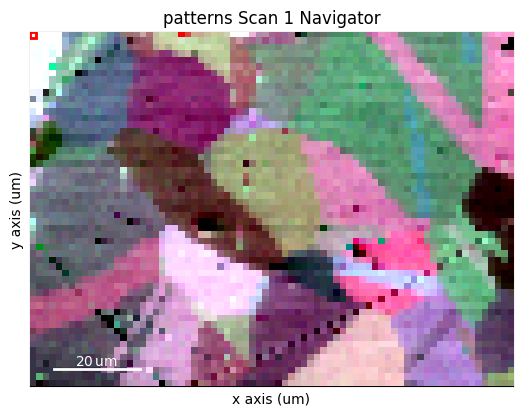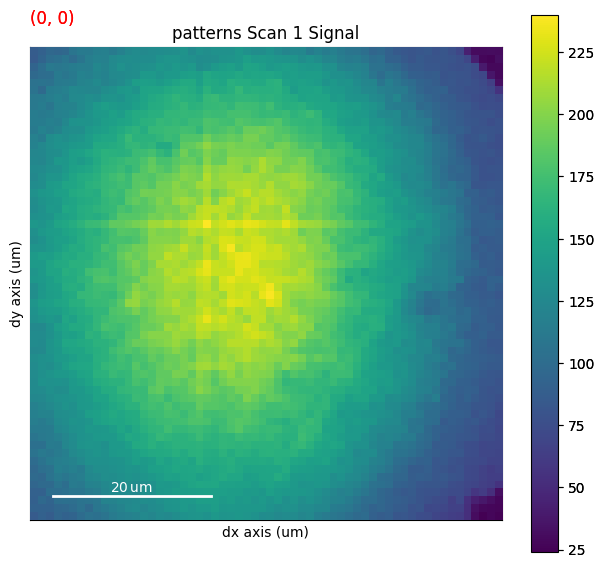## Any image#

Images made into a Signal2D can be used as navigators. For example a quality metric map such as the image quality map calculated using get_image_quality()

:

s.remove_static_background()
s.remove_dynamic_background()

[########################################] | 100% Completed | 101.55 ms
[########################################] | 100% Completed | 607.85 ms

:

maps_iq = s.get_image_quality()
s_iq = hs.signals.Signal2D(maps_iq)
s.plot(navigator=s_iq)

[########################################] | 100% Completed | 302.44 ms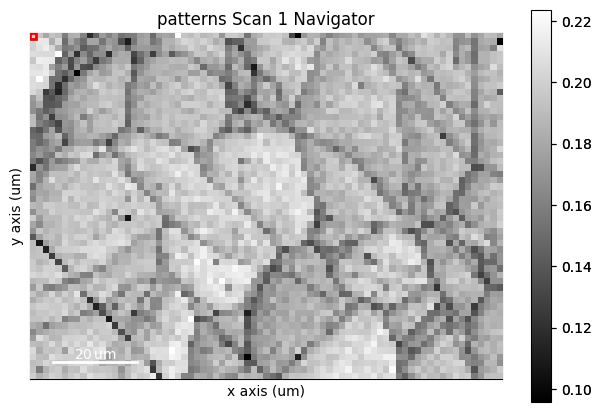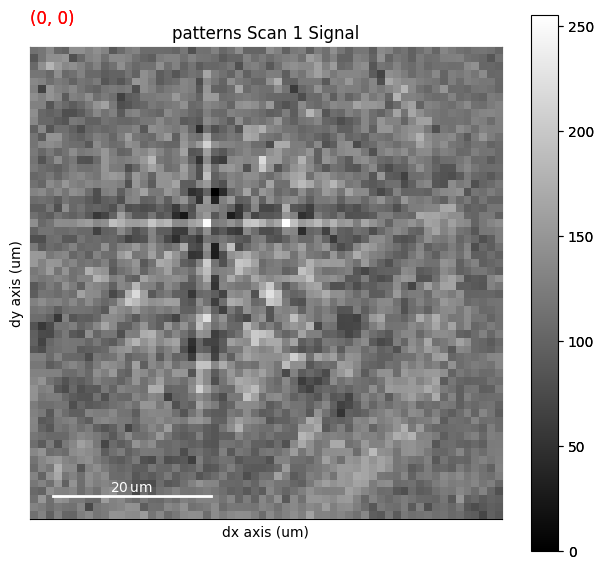We obtain an RGB signal from an RGB image using get_rgb_navigator(). Let’s load an orientation map from dictionary indexing in the pattern matching tutorial

:

maps_ipfz = plt.imread("../_static/image/visualizing_patterns/ni_large_rgb_z.png")
maps_ipfz = maps_ipfz[..., :3]  # Drop the alpha channel
s_ipfz = kp.draw.get_rgb_navigator(maps_ipfz)

s.plot(navigator=s_ipfz, colorbar=False)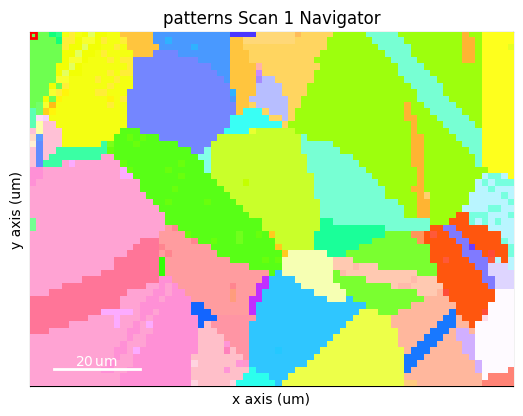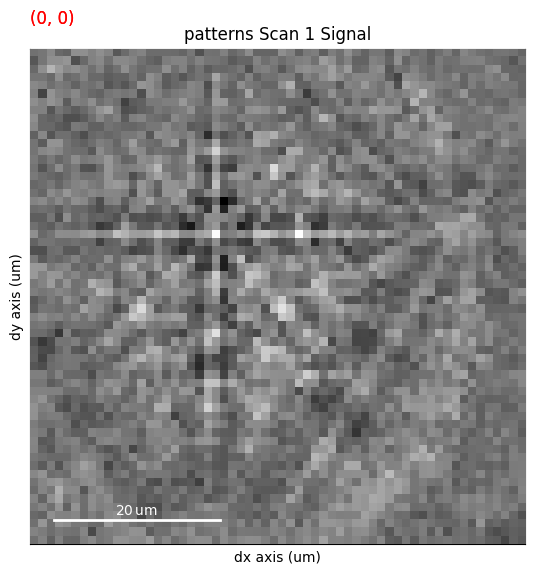By overlaying the image quality map on the RGB image, we can visualize crystal directions within grains and the grain morphology in the same image

:

maps_iq_1d = maps_iq.ravel()  # Flat array required by orix
maps_ipfz_1d = maps_ipfz.reshape(-1, 3)
fig = s.xmap.plot(maps_ipfz_1d, overlay=maps_iq_1d, return_figure=True)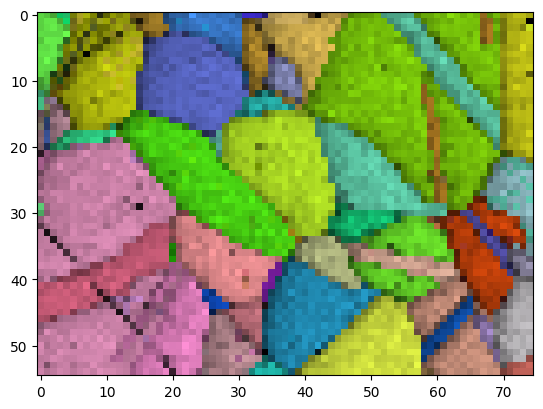By extracting the image array, we can use this map to navigate patterns in

:

maps_ipfz_iq = fig.axes.images.get_array()
s_ipfz_iq = kp.draw.get_rgb_navigator(maps_ipfz_iq)
s.plot(s_ipfz_iq)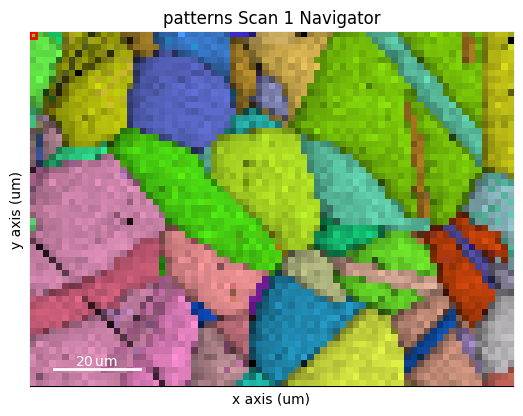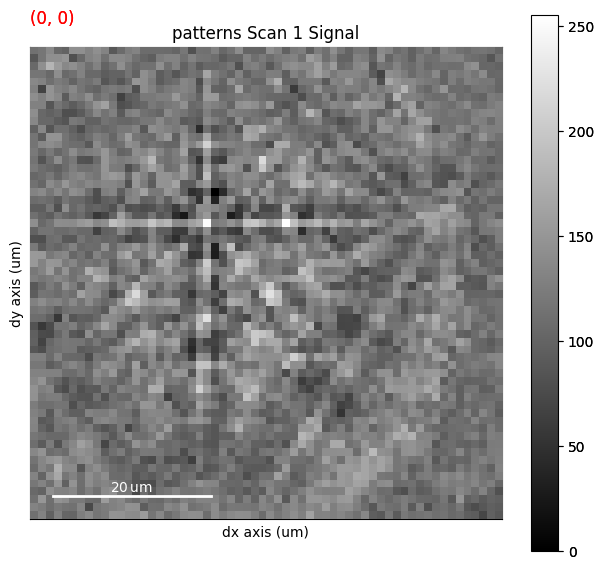## Plot multiple signals#

HyperSpy provides the function plot_signals() to plot multiple signals with the same navigator, as explained in their documentation. This enables e.g. plotting of experimental and best matching simulated patterns side-by-side as a visual inspection of indexing results. See the pattern matching tutorial for a demonstration.

## Plot master patterns#

EBSDMasterPattern signals can be navigated along their energy axis and/or their northern/southern hemisphere. Let’s reload the Nickel master pattern used in the previous section, but this time in the stereographic projection.

:

# Only a single energy, 20 keV
mp_stereo = kp.data.nickel_ebsd_master_pattern_small(
projection="stereographic", hemisphere="both"
)
print(mp_stereo.axes_manager)

<Axes manager, axes: (2|401, 401)>
Name |   size |  index |  offset |   scale |  units
================ | ====== | ====== | ======= | ======= | ======
hemisphere |      2 |      0 |       0 |       1 |
---------------- | ------ | ------ | ------- | ------- | ------
width |    401 |      0 |  -2e+02 |       1 |     px
height |    401 |      0 |  -2e+02 |       1 |     px


As can be seen from the axes manager, the master pattern has two navigation axes, the northern and southern hemispheres, thus, when plotting, we get a navigation slider

:

mp_stereo.plot()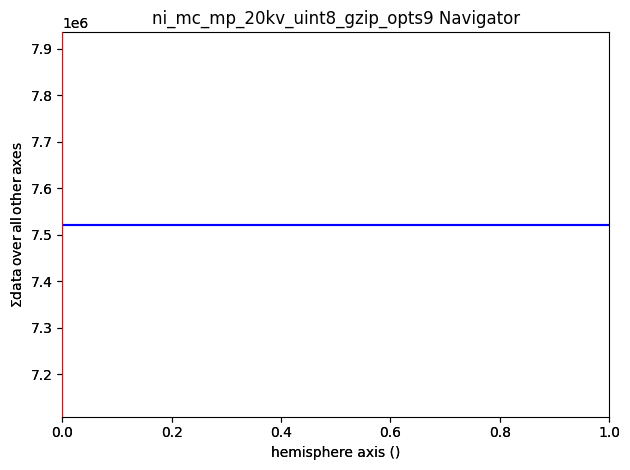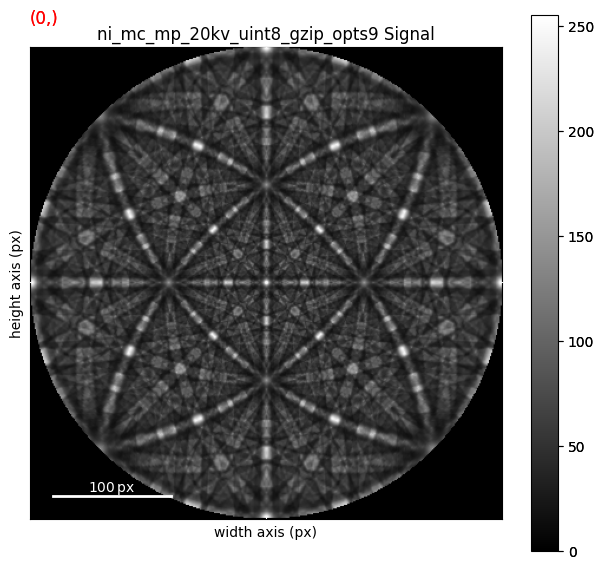We can plot the master pattern on the sphere, provided it is in the stereographic projection and that both hemispheres are loaded if it is non-centrosymmetric, using EBSDMasterPattern.plot_spherical(). The initial orientation of the sphere corresponds to the orientation of the stereographic and Lambert projections.

:

# Interactive!
mp_stereo.plot_spherical(style="points")